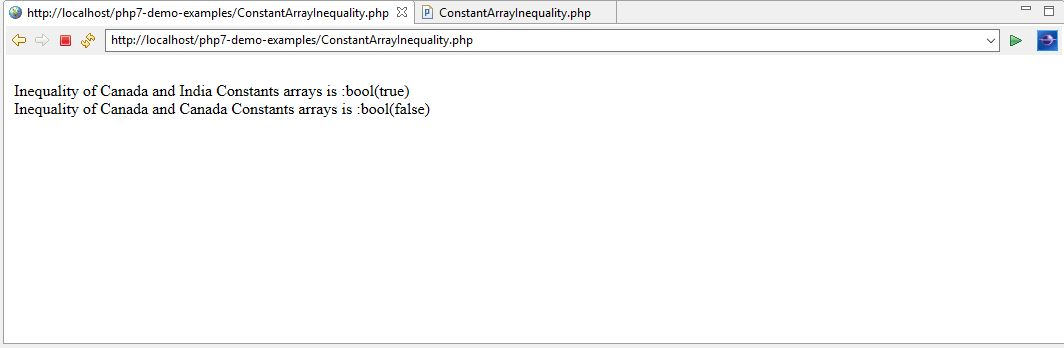# Learn about Constants Array in PHP7

1
3281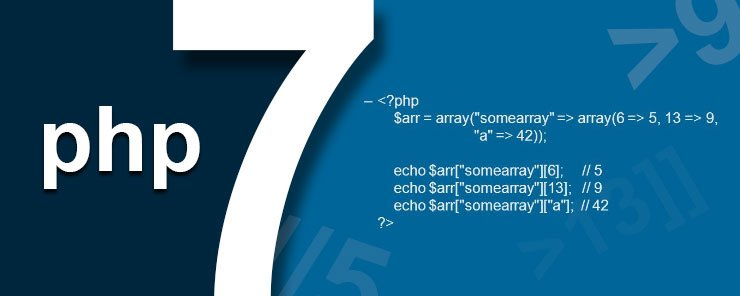In the last chapter, we discussed two new PHP 7 operators – null coalescing and spaceship operators with examples. In this chapter, we are going to discuss about array of constants, which is again one of the many new features added to PHP 7.

Constants Array
Before PHP 7, we were using the const keyword to define an array of constants. PHP 7 has a new feature allowing us to define an array of constants using the ‘define ()’ function as shown below.
Here, the constants array name is Canada and city names such as ‘Calgary’, ‘Montreal’, ‘Ottawa’, ‘Toronto’, and ‘Vancouver’ are the constants. Like any other array, each constant has an index starting from 0 to number of elements minus one. Therefore, element at zero index will be ‘Calgary’ and at index as 1 is ‘Montreal’ and so on.

We are going to learn about constants array in detail with the following example.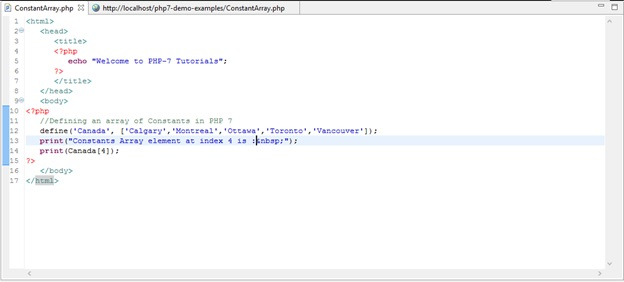Explanation of PHP 7 code
We have used the ‘define ()’ function to declare an array with the name as Canada which has 5 constant values that cannot be modified or deleted.

Output
In the above program, we are printing the value of array at 4th index which has a value ‘Vancouver’.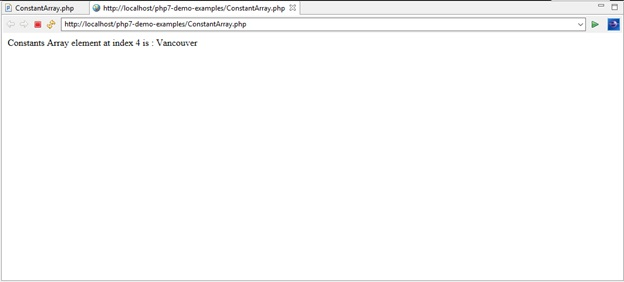Union of Constant Arrays
We use the + operator for joining two arrays. The joining takes place at the index level of the arrays. E.g. ‘a’ and ‘b’ are two arrays. Array ‘a’ has 4 elements and array ‘b’ has 6 elements. Then the combination of these two arrays i.e. \$a + \$b will be equal to first 4 elements of array ‘a’ and then 5th and 6th element of the array ‘b’. This is demonstrated below.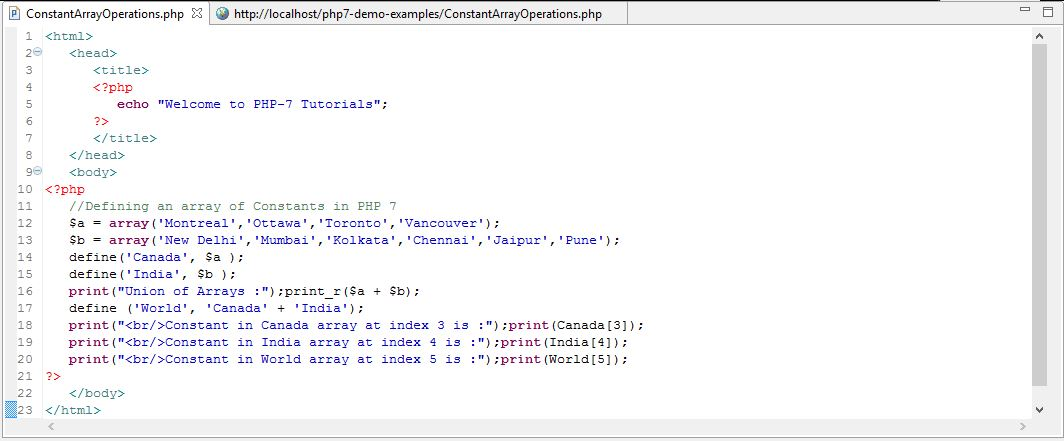Explanation of PHP 7 Code
Here, we first declared two arrays – array ‘a’ and array ‘b’, then we used the define function to create two constants array ‘Canada’ and ‘India’, respectively. Constant array ‘Canada’ has 4 elements namely Canada  = Montreal, Canada  = Ottawa, Canada  = Toronto, Canada  = Vancouver. Similarly constant array ‘India’ has 6 elements namely India  = New Delhi, India  = Mumbai, India  = Kolkata, India  = Chennai, India  = Jaipur, India  = Pune. Now, using define () function feature, we are defining a constants array ‘World’ using + operator which will combine the ‘Canada’ and ‘India’ arrays. After union, World array will have total of 6 elements namely World  = Montreal, World  = Ottawa, World  = Toronto, World  = Vancouver, World  = Jaipur and World  = Pune.

Output: After the union of two constants arrays, the resulted array World has total 6 elements and in the program we are printing elements of Canada , India  and World . Below is the output.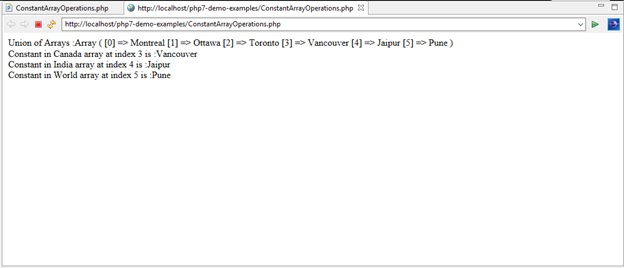Equality of Constants Arrays
We use the == operator to check the equality of two arrays. The equality takes place at the index as well as element value level between the arrays. E.g. ‘a’ and ‘b’ are two arrays. Array ‘a’ has 4 elements and array ‘b’ has 6 elements. Then, the equality check between these two arrays i.e. ‘\$a == \$b‘ will return bool (false) as the array ‘a’ and the array ‘b’ index and corresponding values do not match. This is demonstrated below.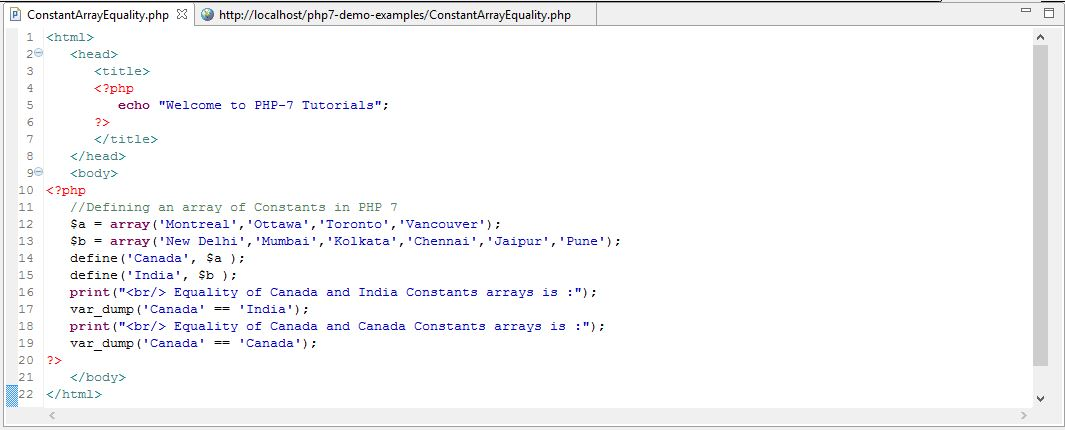Explanation of PHP 7 Code
Here, we used the define function to declare two constants arrays ‘Canada’ and ‘India’ that have 4 and 6 elements, respectively. Therefore, when equality of ‘Canada’ array is checked against ‘India’ array, the equality statement will return bool (false). However, when we compare ‘Canada’ array against ‘Canada’ array, the equality statement will return bool (true) as both arrays are identical.

Output
Below output shows bool (false) when we checked equality of ‘Canada’ array against ‘India’ array and bool (true) when we checked equality of ‘Canada’ array against ‘Canada’ array.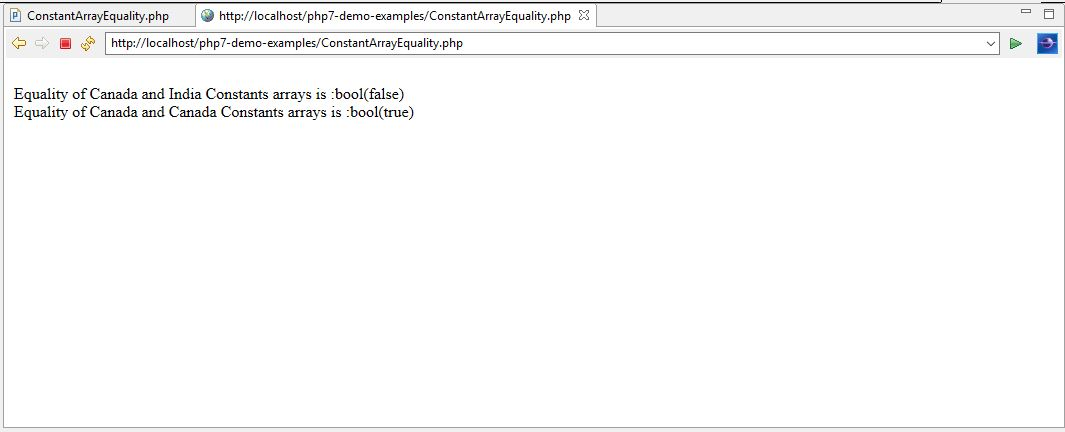Identity of Constants Arrays
We use the === operator to check if the two arrays are identical. If ‘a’ and ‘b’ are two constant arrays that have the same key and value pairs that are in the same order and are of the same types. Then a === b will return true, otherwise false. This is demonstrated below: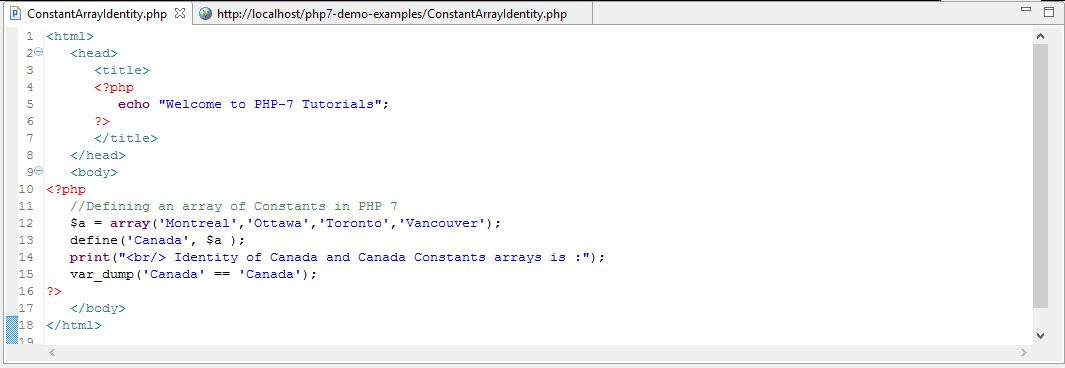Explanation of PHP 7 Code
Here, we used the PHP 7 define function to declare one constants array ‘Canada’ which has 4 elements. When we check for identity of ‘Canada’ array against ‘Canada’ array, the identity statement will return bool (true). However, when we compare ‘Canada’ array against any other different array, the identity statement will return bool (false), as those arrays will be non-identical.

Output: Below output shows bool (true) when we checked identity of ‘Canada’ array against ‘Canada’ array.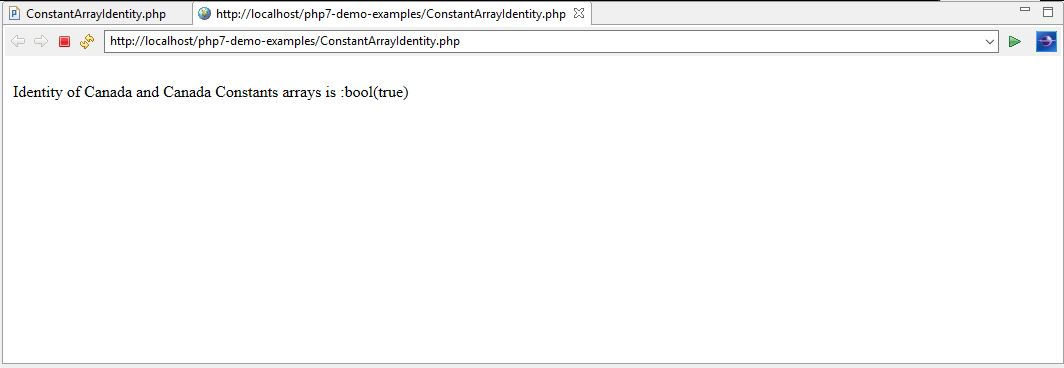Inequality of Constants Arrays
We use != operator to check if the two arrays are equal. The inequality takes place at the index level as well as the value of the elements of the arrays. E.g. ‘a’ and ‘b’ are two arrays. Array ‘a’ has 4 elements and array ‘b’ has 6 elements, then the Inequality check between these two arrays i.e. ‘\$a != \$b‘ will return bool (true) as the array ‘a’ and the array ‘b’ index values do not match. This is demonstrated below.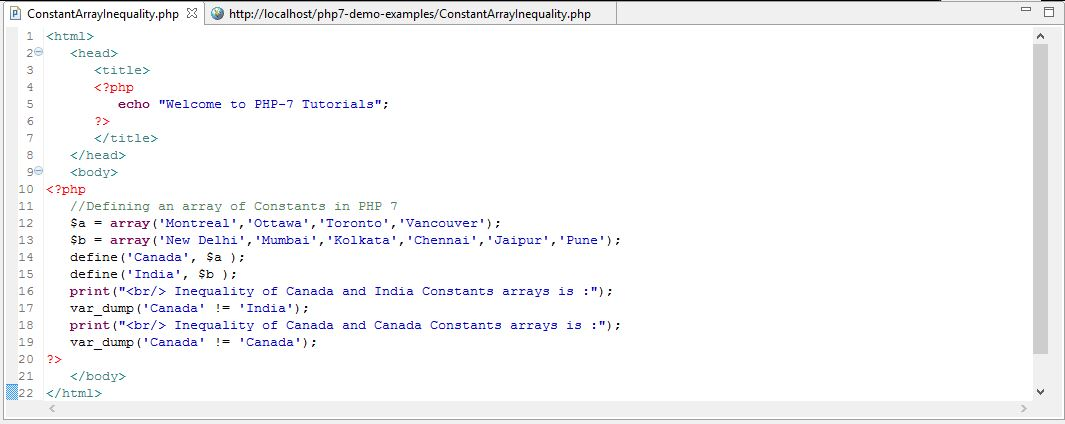Explanation of PHP 7 Code
Here, we used PHP 7 define function to declare two constants arrays namely ‘Canada’ and ‘India’ which have 4 and 6 elements, respectively. Therefore, when inequality of ‘Canada’ array is checked against ‘India’ array, the inequality statement will return bool (true). However, when we compare ‘Canada’ array against ‘Canada’ array, the inequality statement will return bool (false), as both arrays are identical.

Output
The below output shows bool (true) when we checked inequality of ‘Canada’ array against ‘India’ array and bool (false) when we checked Inequality of ‘Canada’ array against ‘Canada’ array.